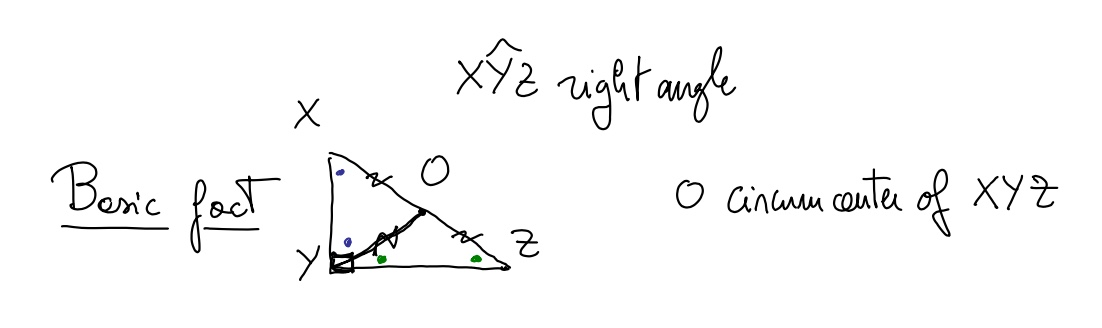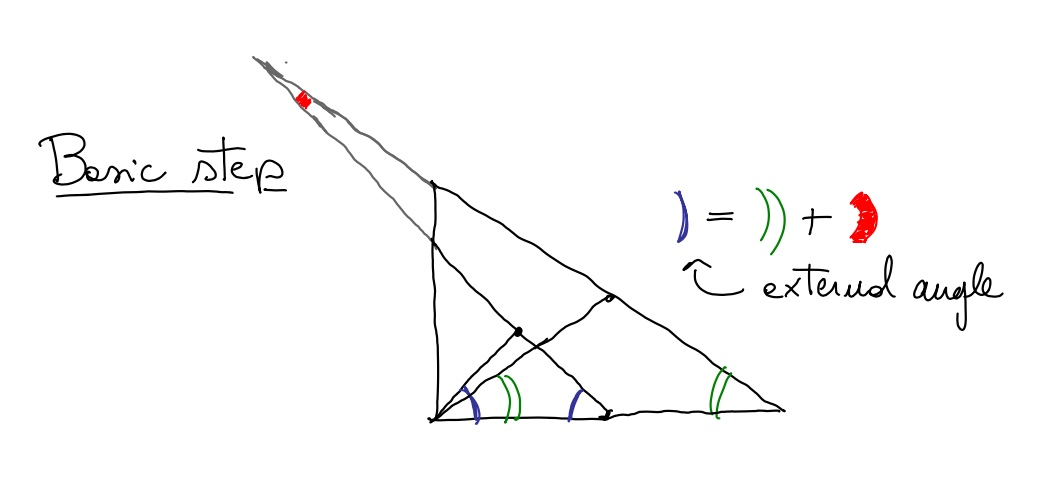# Blog

### Thinking Out Loud – EGMO 2017 Problem 1

EGMO 2017, Problem 1. Let $ABCD$ be a convex quadrilateral with $\widehat{DAB} = \widehat{BCD} = 90^\circ$ and $\widehat{ABC} > \widehat{CDA}$. Let $Q$ and $R$ be points on segments $BC$ and $CD$, respectively, such that line $QR$ intersects lines $AB$ and $AD$ at points $P$ and $S$, respectively. It is given that $PQ = RS$. Let the midpoint of $BD$ be $M$ and the midpoint of $QR$ be $N$. Prove that the points $M, N, A$ and $C$ lie on a circle.

Ok, so, problem one. Should be easy. Oh. Geometry. Ok, well maybe it's... oh, it's not a triangle.
Ok ok ok, like the Guide says, don't panic!
Let's see... we have a quadrilateral with some right angles, a lot of equal segments and we want a cyclic quadrilateral.
Ah, I said "cyclic"... well, the quadrilateral we start with, $ABCD$ that is, has two opposite right angles, by hypothesis, which is the most famous instance of a cyclic quadrilateral. So, good news: we start with $ABCD$ cyclic and we want $AMNC$ cyclic.
A drawing could help...But $M$ and $N$ are not really defined in a good way that relates to the angles of $ABCD$... actually, $N$ is defined in a very peculiar way... oh, wait, $N$ is the midpoint or $QR$, but extending $QR$ to meet $AD$ and $AB$ we obtain $S$ and $P$ such that $SR=PQ$, so, to sum it up, $N$ is the midpoint of $PS$ and, as we already knew, $M$ is the midpoint of $BD$.
Aaaaand we have right angles all around! Look at the picture! $PS$ is the hypothenuse of the right-angled triangle $APS$, $BD$ is the hypothenuse in two right triangles, $ABD$ and $CBD$. Oh, wait, $RQ$ is the hypothenuse  of $CRQ$. We have a pattern here...
The midpoint of the hypothenuse is the circumcenter of the right triangle: this fact allows us to move a lot of angles around!So, let's get some stuff done with $AMNC$... we want to show that it is cyclic. This can be obtained in various ways, but we notice that we have two right-angled triangles with circumcenter $M$, which have the right angle in $A$ and $C$ and two right-angled triangles with circumcenter $N$, which have the right angle in $A$ and $C$. So, it seems reasonable to look at the angles $\widehat{MAN}$ and $\widehat{MCN}$.
For example: let us consider $\widehat{MAN}=\widehat{MAB}-\widehat{PAN}$. In the triangle $BAD$, $MA=MB$, so $\widehat{MAB}=\widehat{ABD}$; in the triangle $PAS$, $NA=NP$, so $\widehat{PAN}=\widehat{APS}$.We end up with
$$\widehat{MAN}=\widehat{ABD}-\widehat{APS}$$
and, if we extend $BD$ and $PS$ and let them intersect each other in $E$, we finally obtain $\widehat{MAN}=\widehat{BEP}$ (by viewing $\widehat{ABD}$ as an external angle of $BEP$).
Now, we know what to do: $\widehat{MCN}=\widehat{NCQ}-\widehat{MCB}=\widehat{RQC}-\widehat{DBC}=\widehat{BEQ}$ (by viewing $\widehat{RQC}$ as an external angle of $BEQ$).
This finishes the proof: as $\widehat{MAN}=\widehat{MCN}$, the quadrilateral $AMNC$ is cyclic.
We are done and happy with our job... uhm wait, what was that annoying hypothesis about angles for? Oh, an inequality... that triggers a pavlovian reaction: I have to look for configuration issues (typical, with cyclic quadrilaterals) .... probably, the inequality guarantees that there is only one configuration possible.
Once we make sure of this last thing, the problem is really over and we can happily go on to problem 2, which won't be geometry...